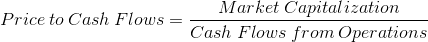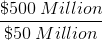Price to Cash FlowsThe formula for the Price to Cash Flows ratio, or P/CF, is a company's market capitalization divided by its cash flows from operations. The numerator, market capitalization, is the total value for all stocks outstanding for a company. Market cap can typically be found with many stock quotes, along with other common stock metrics. If an individual needed to find the number of shares outstanding, this could be found on the company's income statement. The market cap can be calculated using the number of shares outstanding and the price per share.

The denominator, operating cash flows, can be found on the company's cash flow statement. The cash flow statement is reported annually by companies along with other financial statements. The cash flow statement looks at a company's cash transactions for the year. This statement does not include non-cash transactions found on the income statement, such as depreciation and changes in receivables and payables (working capital).

Example of the Price to Cash Flows Formula

An example of calculating the price to cash flows would be to assume that a company has a market cap of \$500 million. If this information wasn't readily available, an individual could calculate this based on a share price of \$25.00 with 20 million shares outstanding. The statement of cash flows shows net operating cash flows of \$50 million. Using these variables in the formula at the top of the page would showwhich would result in a P/CF of 10.

Use of the Price to Cash Flows Formula

The price to cash flows ratio may be used along with or in place of other stock pricing formulas, particularly price to earnings, price to sales, and price to book value.

The distinction between the formula for the P/CF ratio and the price to earnings ratio, is that earnings uses net income found on the income statement, whereas cash flows uses only the cash transactions. The P/CF can be considered a better metric when a company's operations can better illustrate the well-being of a company than bottom line earnings. For example, a company may have negative net earnings due to high depreciation, or large swings in working capital.

The distinction between the formula for the P/CF ratio and the price to sales ratio, is that sales revenues does not look at any expenses, neither costs of goods sold nor operating expenses. If cash flows are negative, one could use sales to make a calculation, however, at the end of the day, negative cash flows is a large issue.

The distinction between the formula for the P/CF ratio and the price to book value ratio, is that the two are likely to be used together instead of an 'either/or' situation. The P/CF ratio, similar to the two other ratios discussed above, looks at how much an investor is paying in relation to how well the company is earning. The P/BV, on the other hand, looks more at price of the stock, and on a larger level, the market cap, relative to book value of a company. Both may be used for valuation purposes, but the methods are unrelated.

New to Finance?